Home Python Python For Loop: The Definitive Guide With Video Tutorial

# Python For Loop: The Definitive Guide With Video Tutorial

0

Loops are one of the first beginning blocks that you need to understand in order to actually start the process of automation in any programming language. Mastery over loops is a must for anyone aspiring to be a great programmer.

The concept of loops is consistent across programming languages. Hence, you learn loops once and it would be useful for you across different programming languages.

Hello Guys,

First, let us start this article a very simple and very obvious question and that question is:

Contents

### What do we mean by loops in general?

Loops as the name suggest meaning some repetition. So, we know it has to do with repetition. So, how does it all play?

You make a circle of a particular radius says 3cm. Then, you make another circle for the radius 4cm, and then the third time you drew one another circle which was of radius 1cm.

In all of the above three statements, you were essentially drawing a circle again and again with some different radius. So, as a whole, I can replace the above three statement with this one single statement:

You make three circles of radius 3cm, 4cm, and finally of 1cm.

The above example really captures what we mean by looping. Essentially looping means something that repeats itself.

Now, how does the concept of looping is used in programming languages?

The programming languages were created to make the life of humans easier. We somehow wanted to automate some of our repetitive tasks. If you notice yourself in a day, then you would know that on a usual day many times you would do something that will be a good use case for looping.

For example, almost everyone, who uses a laptop, the moment someone powers their laptop on, the first action they perform is usually to refresh the system. This task is done almost daily without realizing that it cannot be repeated using loops.

So, loops are essentially about automating something that is similar in nature. Any sort of variation in that similarly can easily remove the task from the list of tasks that can be automated.

You open a file and you make folders, all these tasks can have some sort of shortcuts associated with it. In fact, we do have some shortcuts associated with creating a new folder.

We press Control + shift + enter to create a new folder and when we do that there must be some sort of backed programming language that is run and it automatically creates that folder for you. So, here you see an example of automation.

Similarly, we can have a task for going over a list of names and adding some sort of greeting message to each name, and if we do that manually, it would take some time but if we choose to do that using the for loop, then it would take a fraction of a millisecond.

So, what is the summary of the discussion of the loop that we have just had?

Loops are essentially a way of repeating some tasks that can be repeated.

## Loops in Python

Now, let us look at the loops in the context of Python programming. The above discussion was a much general discussion which can be applied to almost any programming language.

Python provides two ways of making use of loops:-

1. For loops
2. While loops.

For loops are what we will be discussing in great detail in this article.

Let us take a very simple scenario where loops can be used.

Let us say, we have a list of names and we would like to make another list with the corresponding greeting for each name.

Now, how can we go about solving this problem with python? 🤔

Let us look at one way (which is a tedious way) and then after talking about this way, we will then talk about the loops and see how loops can be used to automate this simple process.

First, let us open IDLE and create a new script with the following code in it: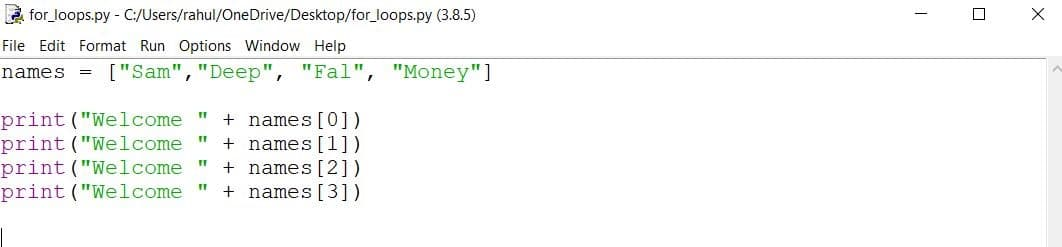So, what we do in the above script is first we declare the list of names that we want to add the greeting to and then one by one by accessing each list’s element and then append Welcome in the front of it.

So, after we run the script, we get the following output:-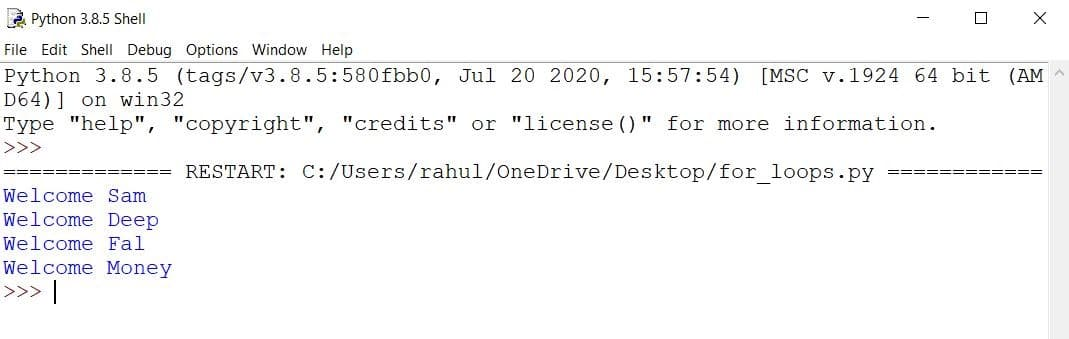This process involves a lot of copy-pasting and making some small tweaks to each pasted statement. The way around this repetitive code is loops.

Let us first look at how we will solve the above issue of repetitive code and then we will look at the syntax of the for loop in a little bit deep manner.

The following is the for loop that prints the same output that we have seen earlier…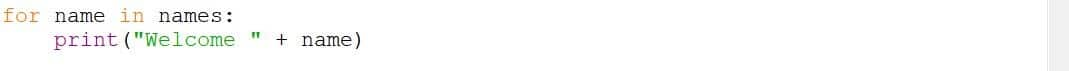So, now let us look at the output of the above code.From the above output on the IDLE, we can clearly see that the output is just the same as the previous output but with a lot less code.

### Syntax of For loop in Python

Okay, now that we know that the for loop can indeed solve the problem, we need to understand the syntax of the for loop.

Instead of calling the for loop “for loop”, we should rather call it “for – in” loop. Because in python wherever it comes, it will automatically come.

So, loops in python are used to iterate through an iterable data structure. An iterable data structure is a data structure in python which can be iterated upon using a for loop.

For such data structure, we have some elements in it. So, when we say for a variable in some_iteratable, what we are saying is that for each variable in that iterable (list in the given example), do something with the current_assignment (variable in this case).

Let us understand this with the above example piece by piece. So, we have a list that stores some names. The names in it are called items.

When we start the for loop by saying “for name in names”, we are declaring a temporary variable called name to store the first name which is “Sam” in this case, then we enter the loop where we do something with this “Sam” which is currently assigned to the temporary variable “name” and after that operation of that temporary variable has been completed, then the loop again starts that temporary variable initialization but this time it moves to the next eligible item in the iterator.

Then, the same operation is executed on the next item in the iterator and in this way, this cycle goes on until all the items are exhausted.

So, this is how the loop works in Python. Now, let us loop at some more examples of loops below.

#### Example. 1 Loops for obtaining the sum of numbers in a list.Here, we first declared a list of numbers and then looped through that list to compute the sum of numbers in that list.

#### Example. 2 Loops with range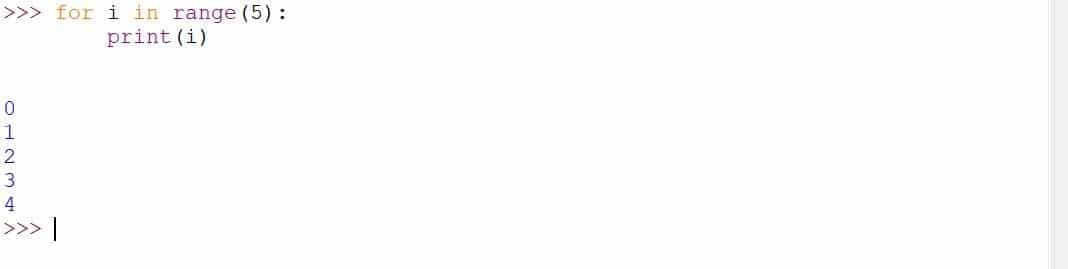Here, we have used another iterator (apart from the list example that we have been looking so far) to kind of loop through that range to generate and print a list of numbers from 0 to 4.

#### Break and Continue Statement

Okay, now that we have talked about how to work with loops in python, let us now look at some important aspects and concepts of loops that would be a good addition in your looping skills.

First, let us look at how to use the “break” statement with a loop. So, what is a break statement in loop? A break statement in loop is a statement which enables us to come out of loop after a certain condition has been fulfilled. Now, why is this necessary?

Loops might run forever if they are told to but soon our computers will crash if they are continued to let run.

For Loops will run as long as the elements in the iterable are not exhausted. But if we want to come out of loop before a certain iteration.

Welcome to the world of “break” statements with for loops. This enables us to add an if statement with the loops which on being true, will make us come out of the loop. So, let us look at a very simple example.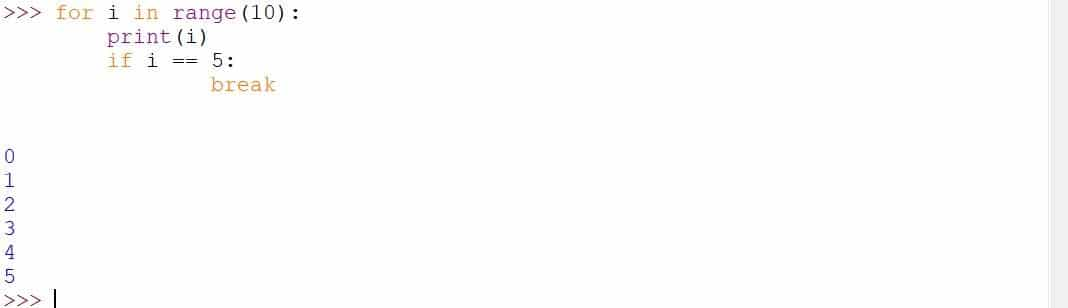Take a good look at the code above and you will notice a few things. The length of the iterable is 10 but the print statement not executed only for 6 times.

Why didn’t it print other values like 6, 7, 8, 9. The reason for this is a break statement. As soon as the value of 5 is achieved by i, the break statement gets executed and it takes us out of the loop.

Okay, now that we understand how this thing works, we need to understand where can thus be actually used? So, a use case of anything in programming is really important.

One of the best use cases of this kind of breaking out of the loop is game. In games, there is always a concept of loops. Loops allow each frame to get displayed in a continuous fashion. If a certain event happens within the game (for example player click the quit button), the game would be closed.

#### Continue Statement

After talking about break statement in for loops, we now need to talk about continue statement. Let us first understand where we might find it useful to use a continue statement.

As the name suggests, it continues (skips ) the current iteration for a certain iteration whereas the break will simply breaks out of the loop.

Hence, in this manner, the continue statement does not cause the loop to get closed and broken but instead the current iteration is just skipped and anything after that if-continue statement inside the fir loop will not get executed.

Now, let us take the same example of game playing and let us try to find the use case of “continue” in that context. So, in the game, we always have some moves which do not cause the heath loss to the player but those moves are very rare.

For those moves, the continue statement can be used. So, we could have a for loop for health loss which on a constant basis, takes out some health of the player but on a certain condition, that loop’s statement might not get executed (or just skipped).

Now, let us look at an example of a “continue” statement where all the values ranging from 0 to 9 are printed except for the value 6.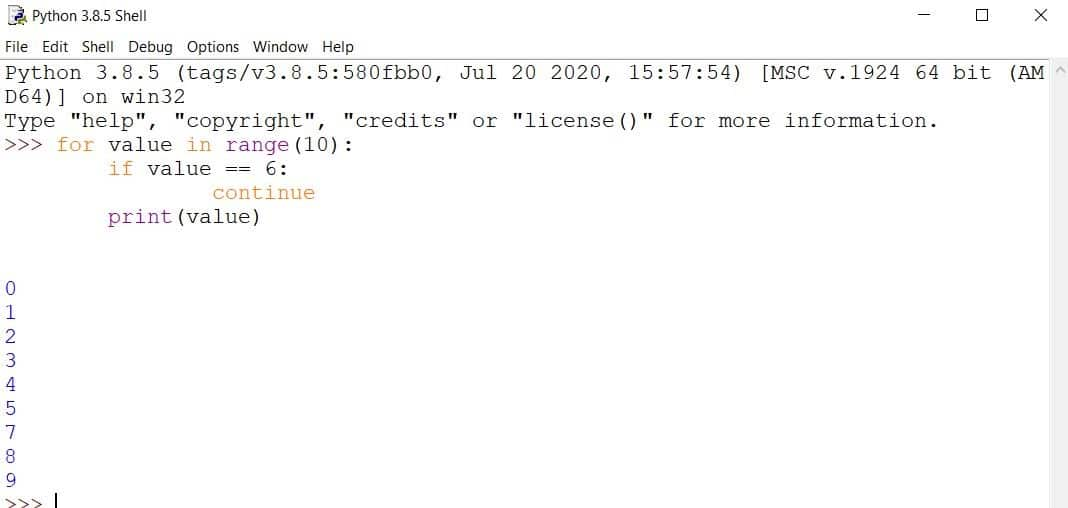In the above code result, you might notice that 6 is missing but all the other numbers are clearly written. The reason 6 is missing is that there is a condition that says if the value is 6, then we will skip that iteration, and hence when that is true, all the lines that follow that will be ignored for that particular iteration only.

#### Nested loops (Loops inside loops)

Consider you need to create a matrix of order 3 * 2 using lists with some elements say 1. How would you go about it? One way is just to manually type the values but there is a one more elegant way and that way is by using nested loops.

Let me first show you an example and then we will go through that example to understand how the nested loops are created and how they work.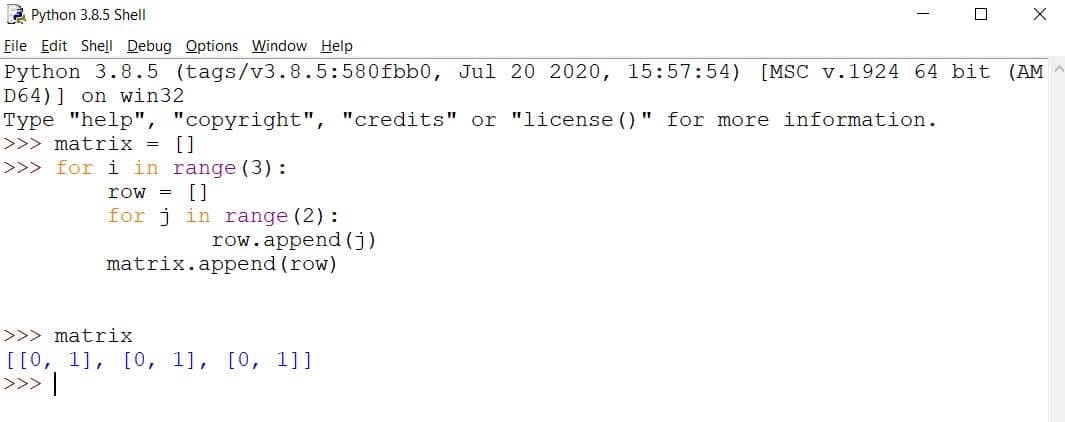I want all of you to pay attention to what the above piece of code is doing. Because it gets a little tricky for a beginner programmer to actually understand how the nested looping works and it requires a good amount of discussion.

So, let us one by one understand each piece of code.

First, we start by making an empty list called “matrix”. Then we start a for loop with the iterator object range for 3 times and in each of these times, we have first created an empty list called row and then again used a loop with range for 2 times to append all the values in this row.

Once, a row has been created, then we come out of this inside loop and append the newly created row as a whole to the matrix list.

Hence, this matrix is a list of lists.

Here, you need to note that for each of the outside loops, first the inside loop will be completely executed and then only we will move on to the next iteration of the outer loop.

#### One more example for nested loops

To understand this point once again, let me take one more example.

Let us say I have two lists. One list is a name list and another list is the list of different types of greeting that can be used. Then, my task is to use nested loops to print each name with each of the greeting methods.

Let us first look at the code to do that.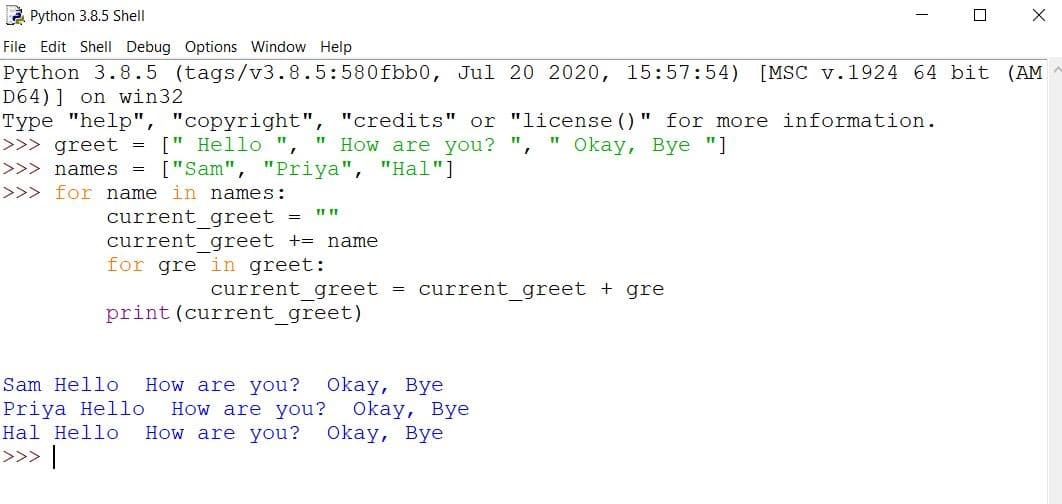Obviously the above greeting is not one of the best but it drives home the point which I want to give. Okay. Now, let us understand the above code piece by piece.

First, let us define the two lists “names” and “gree”. But notice that the greetings in the greet list have been put in a special way. Both at the beginning and at the end of the greeting, we have introduced the space so that there is no difficulty in the printing in the for loop.

Then, we have looped through the names list and appended the name to the current greeting which has been initialized as an empty string just inside the first loop.

Then, we loop through the second greet list and add each of the greetings to the current greeting  and finally after coming out of this (nested) loop, we print the current greet to the console.

So, this was a small discussion on nested loops.

#### Other iterators

Remember, while defining the for loop, we mentioned that for loops can only be used to loop through some data structures which are of type iterator. So, far we have looked at two of such iterators list and range.

Now, let us look at some of the other iterators which can be used with for loops.

One such iterator with which we can use for loops is called “string”. String is a data structure in python composed of only characters. So, for string iterators, each item is a single character. Let us demonstrate this point below: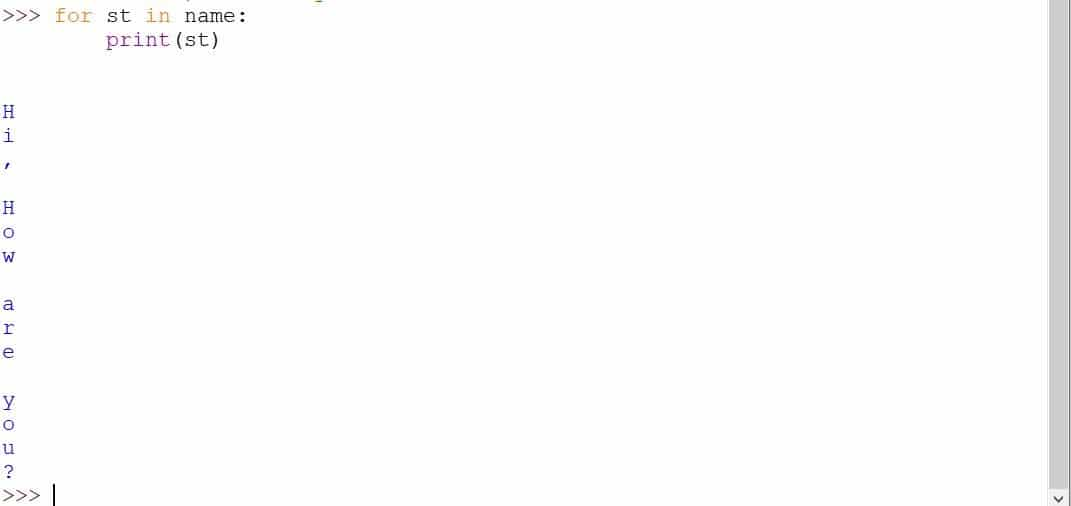Here, first we have declared the name of the string as “How are you?” and notice when I started I used “for in” loop on the string name, then it just printed the individual characters.

#### Using Python for loops with Dictionary

So, by default, a dictionary is not an iterator but their keys and values are. So, to use for loops with a dictionary, we need to first get access to their keys or values.

Let us first define a dictionary and loop through their keys and print their keys one by one using the for loop.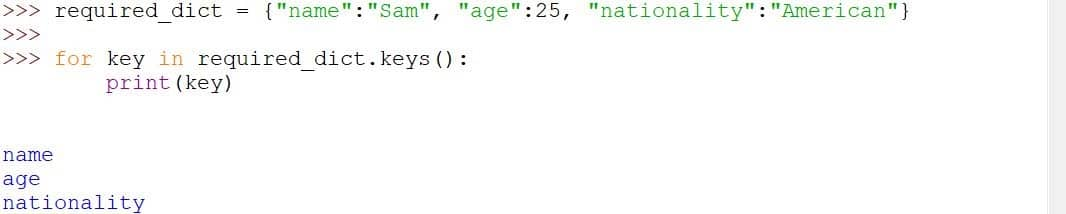Here, we used the dictionary’s keys() method to get an iterator and then looped through it to print it.

Then, let us print the values corresponding to each key by accessing its values. It is quite easy and follows the same pattern as above.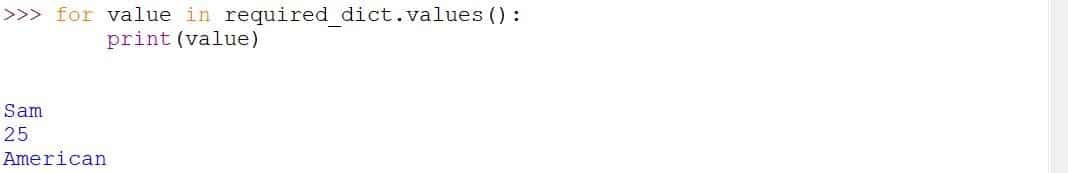Again here also, we got the values from the values method on the list to get an iterator and then looped through it.

Finally, let us use the items method to get an access list of tuples where each tuple will just be a key-value pair of the dictionary.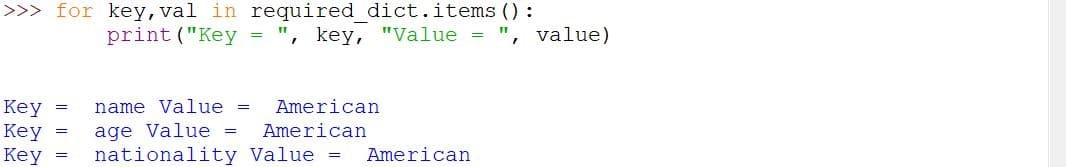Here, unlike in the above two scenarios, we have accessed a list of tuples where each tuple represents the key-value pair and then we have used the print on these two values.

#### Using Else in the for loop

One final thing that I want to discuss before closing off this article is the concept of else statements in the for loops. Else statement is usually used with if statement to cover that condition for which if the condition was not met.

So, there is an if statement which is tested first, and if that statement is tested false, then python looks for the else statement, and that code inside the else statement will be executed.

Now, how can we use else with for loops? Generally, it is done to print some information about the completion of the loop. After the for loop has been executed completely (after all elements in the corresponding iterator has been exhausted), then the else statement is generally executed.

Let us take an example to demonstrate this concept.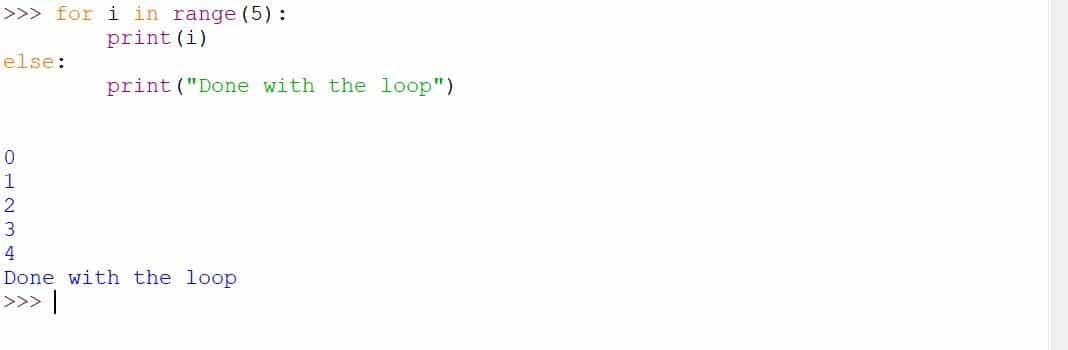In the above code block, we start a loop in the range of values from 0 to 5 and then print those values and after the loop statement ends, we also have an else statement that prints the message that the loop was successful.

So, I hope you have been able to understand the concept of else statements with loops.

Problem.1 Use a “for” loop to generate a function that takes an input n and generates a table for that number from 1 to 10.

Problem. 2 Use a “for” loop to print all the letters that are there in an alphabet.

Problem. 3 Use a “for” loop to print all the digits that are there in the number system.

Problem. 4 Use a “for” loop to make a function that takes two inputs (number and power) and computes the number raised to the power of the “power” argument. This function should be generated and uses a for a loop.

There is already a function called “pow” but you are not allowed to use that. You need to complete this exercise using loops only.

Problem. 5 Create a list of strings and join all of those in space using for loop. Do not use the inbuilt function for that.

Okay, so, I hope you will attempt these problems on your own. I also hope that you have been able to follow through whatever I discussed in this lecture, and with that said, I will stop right here and encourage all of you to explore more concepts for loops. Also, try finding some more problems in this topic but with a different domain.

Now it’s time to explore our Python For Loop video tutorial.